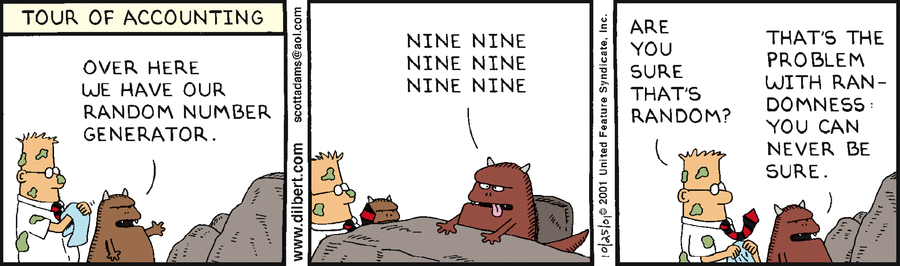# Function - (Non) Deterministic - Predictable

All functions are deterministic or non-deterministic:

• Deterministic functions always return the same result any time they are called with a specific set of input values. If you give them the same set of instructions they always end up with the same result.
• Non-deterministic functions may return different results each time they are called with a specific set of input values.

Whether a function is deterministic or non-deterministic is called the determinism of the function.

If for the same input, the result is unique, the function is deterministic, otherwise non-deterministic.

## Non-deterministic to deterministic

A Non-deterministic SQL function can be made deterministic by ordering the result set on a unique key.

## Example

### Deterministic

The DATEADD built-in function is because it always returns the same result for any given set of argument values for its three parameters.

### Nondeterministic

GETDATE is not deterministic because it is always invoked with the same argument, yet the value it returns changes each time it is executed.

When a function is non-deterministic, there is some kind of randomness.

## Documentation / Reference

Recommended Pages(Function | Operator | Map | Mapping | Transformation | Method | Rule | Task | Subroutine)

Section computable function. A function is a callable unit that may be called: a procedure, a subrontine a routine, a method (belong to an objectmacrocomputablalgorithreusable blocargumentdevelopment...(Function|Operator) - Algebraic (Laws|properties) - Axioms

Property of function and operator Continuous SmoothNumber - Pseudo-random Numbers

Pseudo-random numbers is a sequence of numbers that is predictable if you know the seed. Because true randomness is unpredictable, this is called pseudo randomness (If you know the seed, you can predict...Number - Random (Stochastic|Independent) or (Balanced)

Think of randomness as a lack of pattern. Something random should be unpredictable. We shouldn’t be able to predict the next value of the sequence The degree to which a system has no pattern is known...Oracle Database - SQL - First Value Analytic function

FIRST_VALUE is an non-deterministic analytic function. It returns the first value in an ordered set of values. If the first value in the set is null, then the function returns NULL unless you specify...Oracle Database - SQL - ROW_NUMBER function

ROW_NUMBER is an non-deterministic analytic function. The function assigns a sequential unique number: to each row to which it is applied (either each row in the partition or each row returned by the...Software Design - Predictable